# Biharmonic function

A functionof real variables, defined in a domainof the Euclidean space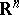,, with continuous partial derivatives up to the fourth order inclusive, that satisfies inthe equationwhereis the Laplace operator. This equation is known as the biharmonic equation. The class of biharmonic functions includes the class of harmonic functions and is a subclass of the class of poly-harmonic functions (cf. Harmonic function; Poly-harmonic function). Each biharmonic function is an analytic function of the coordinates.

From the point of view of practical applications the biharmonic functions in two variables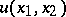are the most important. Such biharmonic functions can be represented using harmonic functions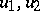or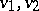, in the form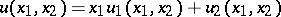or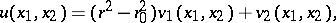where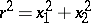, while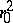is a constant. The main boundary value problem for biharmonic functions is the following: To find a biharmonic function in the domainthat is continuous together with its first-order derivatives in the closed domainand satisfies on the boundarythe conditions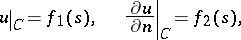(*)

where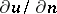is the derivative with respect to the normal on, and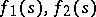are given continuous functions of the arc lengthon the contour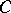. The above representations of biharmonic functions give the solution of problem (*) in an explicit form for the case of the disc, starting from the Poisson integral for harmonic functions .

Biharmonic functions in two variables may also be represented as follows: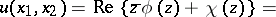using two analytic functions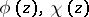of the complex variable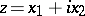. This representation makes it possible to reduce the boundary value problem (*) for an arbitrary domainto a system of boundary value problems for analytic functions, for which a method of solving was developed in detail by G.V. Kolosov and N.I. Muskhelishvili. This method was developed while solving various planar problems of elasticity theory (cf. Elasticity theory, planar problem of), in which the main biharmonic function is the stress function or the Airy function ,  (cf. Airy functions).